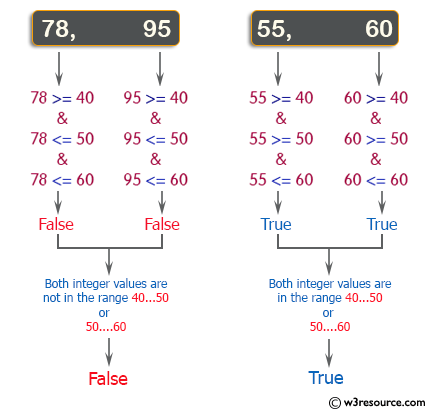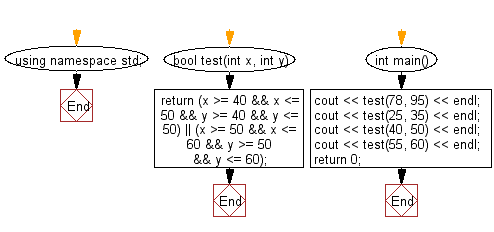﻿ C++ : Check if two given integers are in specific range# C++ Exercises: Check whether two given integers are in the range 40..50 inclusive, or they are both in the range 50..60 inclusive

## C++ Basic Algorithm: Exercise-20 with Solution

Write a C++ program to check whether two given integers are in the range 40..50 inclusive, or they are both in the range 50..60 inclusive.

Sample Solution:

C++ Code :

``````#include <iostream>

using namespace std;

bool test(int x, int y)
{
return (x >= 40 && x <= 50 && y >= 40 && y <= 50) || (x >= 50 && x <= 60 && y >= 50 && y <= 60);
}

int main()
{
cout << test(78, 95) << endl;
cout << test(25, 35) << endl;
cout << test(40, 50) << endl;
cout << test(55, 60) << endl;
return 0;
}
``````

Sample Output:

```0
0
1
1
```

Pictorial Presentation:Flowchart:C++ Code Editor: